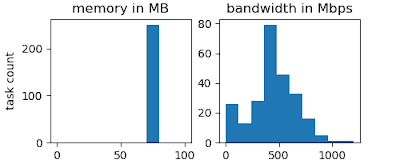## Friday, August 14, 2020

### Resource usage histograms for Work Queue using python's pandas+matplotlib

Work Queue is a framework to write and execute master-worker applications. A master process that can be written in python, perl, or C generates the tasks that then can be remotely executed by worker processes. You can learn more about Work Queue here.

Work Queue can automatically measure the resources, such as cores, memory, disk, and network bandwidth, used by each task. In python, this is enabled as:

```import work_queue as wq
q = wq.WorkQueue(port=9123)
q.enable_monitoring()```

The resources measured are available as part of the task structure:

```# wait for 5 seconds for a task to finish
t = q.wait(5)
if t:
print("Task {id} measured memory: {memory} MB" ```
```            .format(id=t.id, memory=t.resources_measured.memory))
```

The resources measured are also written to Work Queue's transaction log. This log can be enabled when declaring the master's queue:

```import work_queue as wq
q = wq.WorkQueue(port=9123, transactions_log='my_wq_trans.log')
q.enable_monitoring()```

This log is also generated by Makeflow when using Work Queue as a batch system (-Twq).

The resource information per task appears as a json object in the transactions marked as DONE end-state exit-code resource-exhausted resources-measured. Here is an example of how a DONE transaction looks like:

`1595431788501342 10489 TASK 1 DONE SUCCESS  0  {} {"cores": 2, ...}`

With a regular expression incantation, we can extract the resource information into python's pandas. Say, for example, that we are interested in the memory and bandwidth distribution among the executed tasks. We can read these resources as follows:

```import json
import re
import pandas as pd
import matplotlib.pyplot as plt

# the list of the resources we are interested in
resources = 'memory bandwidth'.split()
df = pd.DataFrame(columns=resources)

input_file = 'my_wq_trans.log'

with open(input_file) as input:
for line in input:
# timestamp master-pid TASK id (continue next line)```
```        # DONE SUCCESS exit-code exceeded measured
```                     'DONE\s+SUCCESS\s+0\s+{}\s+({.*})\s*\$', line)
if not m:
continue

# the resources are captured in the first (only pair```
```        # of parentheses) group used:

# append the new resources to the panda's data frame.```
`        # Resources are represented in a json array as`
```        # [value, "unit", such as [1000, "MB"],
# so we only take the first element of the array:
df.loc[len(df)] = list(s[r] for r in resources)
```

For a quick view, we can directly use panda's histogram method:

```df.hist()
plt.show()```
` `
` `

However, we can use matplotlib's facilities for subfigures and add titles,
units, etc. to the histograms:

```# size width x height in inches
fig = plt.figure(figsize=(5,2))

# 1 row, 2 columns, 1st figure of the array
mem = plt.subplot(121)
mem.set_title('memory in MB')
mem.hist(df['memory'], range=(0,100))

# 1 row, 2 columns, 2nd figure of the array
mbp = plt.subplot(122)
mbp.set_title('bandwidth in Mbps')
mbp.hist(df['bandwidth'], range=(0,1200))

fig.savefig(input_file + '.png') ```
` `
``
` `
` `
` `
`(credit: Python code highlight generated by: http://hilite.me)`
` `